### Mechanical Aptitude 2 - Pulleys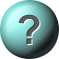1.    How much rope would have to be pulled out of the pulley to raise the object 5 cm?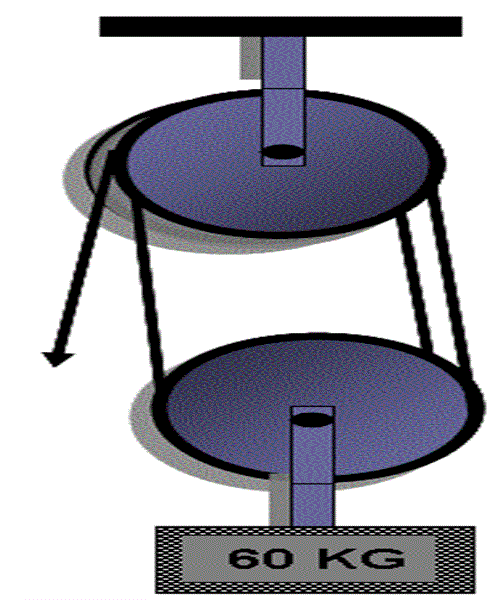5 cm30 cm15 cm45 cm2.    How much rope would have to be pulled out of the pulley to raise the object 5 cm?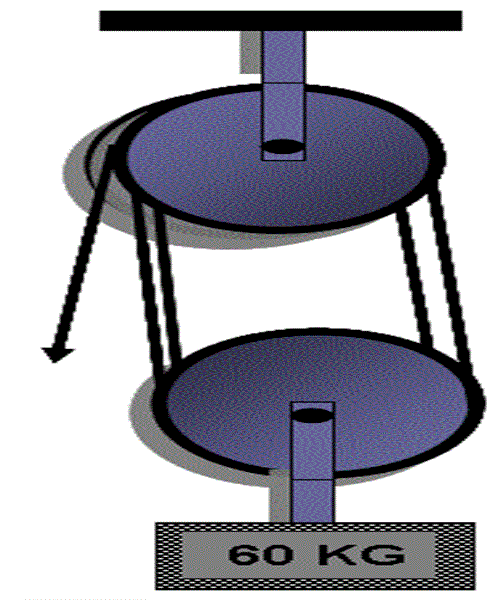30 cm40 cm20 cm25 cm3.    How much force will be required to lift 20 KG weight in the given diagramme?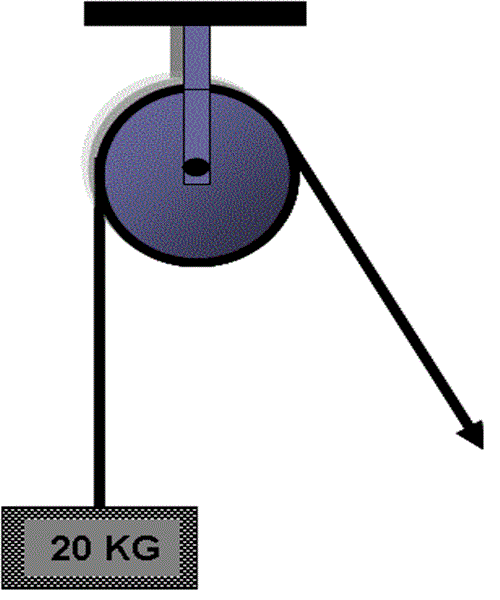25 KG40 KG20 KG5 KG4.    Which weight requires the least force to move?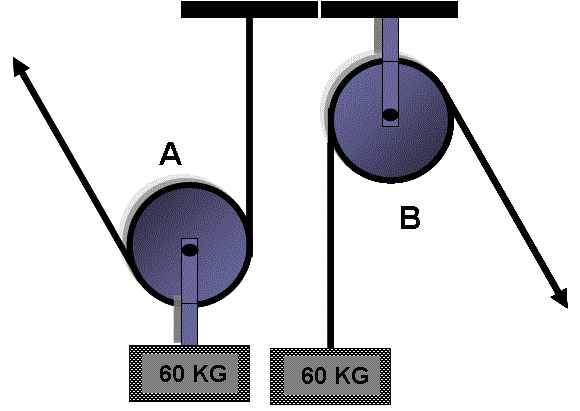Equal Force required in case of weight A and BWeight BWeight A5.    How much force will be required to lift 60 KG weight in the given diagramme?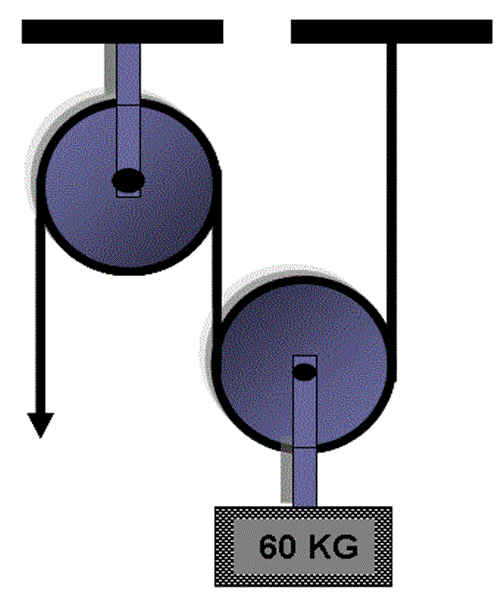15 KG60 KG 6120 KG30 KG6.    How much force will be required to lift 20 KG weight in the given diagramme?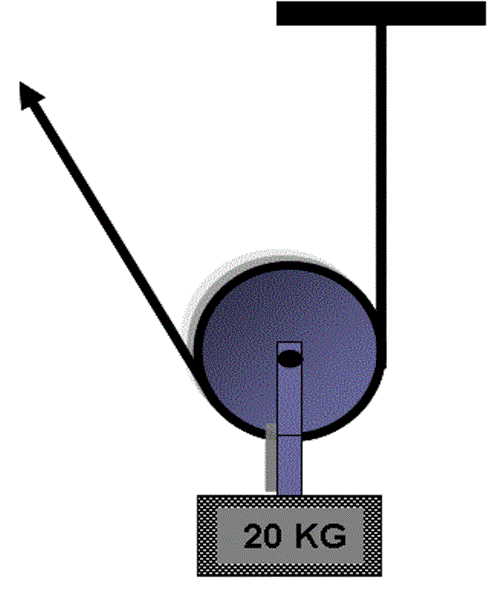20 KG5 KG10 KG25 KG

This is more feedback!
This is the feedback!